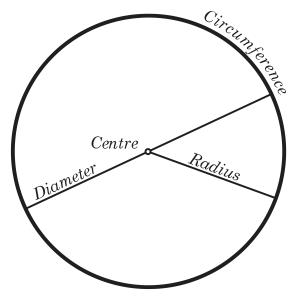•• # Area of a Circle Diameter Formula

The area of a circle diameter formula is A = pi x r2. If you know the circle diameter, you can get the radius by dividing the diameter in two. Next you have to square the radius by multiplying itself. Multiply the result by pi to get the area.

### Defining a CircleThis is the distance around a circle. The diameter is the distance across it. The circumference ratio to the diameter is expressed in pi. A circle’s diameter is double the length of its radius. A circle’s area can be expressed as the number of square units that is within it.

Assume every square in the circle measure 1 cm2; to get the area, just count all the squares. If the circle has 28.26 squares, the entire area would be 28.26 cm2.

### Formulas Used in Circles

Because a circle’s diameter is double its radius, it can be expressed as diameter = 2 x radius. Circumference is pi times the diameter. This is expressed as circumference = pi x diameter. These figures are also true: circumference = 2 x pi x radius and circumference / diameter = pi.

### Names and Terminology

Aside from radius, diameter and circumference, other names are used. A chord is a line that goes from a point in the circle to another line in the circumference. The chord becomes a diameter if the line goes through the middle. A line is called a tangent if it just touches the circle while going through. An arc refers to a part of the circumference.

### Slices

There are two slices in a circle; the segment and pizza slice. A segment slice is created by a chord. The pizza slice is also known as a sector. The most common sectors are the semicircle and quadrant. A quadrant is one quarter of a circle. A semicircle is one half of a circle.

### Other Information

The word circle comes from kirkos, a Greek word meaning “bend”. Studies show that the circle has been known since the dawn of civilization. Knowledge of circles probably came from observing the Sun, Moon and other natural formation.

This information became the foundation for the wheel. This led to the invention of gears and other mechanical objects. This helped in the development of science and mathematics too.

While the area of a circle diameter can be expressed as A = pi x r2, in diameter the formula is different. It is A = (pi/4) x D2.